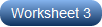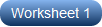# Dividing Decimals by Powers of Ten Worksheets

Increase your practice manifold with our printable dividing decimals by powers of ten worksheets. Let grade 5 and grade 6 students comprehend that dividing a number by the power of ten decreases its value. Children also recognize that in a multi-digit number, a digit in the ones place represents 10 times as much as it represents in the place to its right and 1/10 of what it represents in the place to its left. These exercise pdfs provides students the much needed practice in the most important part of dividing decimals, which is moving the decimal point to the left as many places as the power of ten they are divided by and the appropriate number of zeros to be added in the quotient. Cross check your solutions with our answer key. Work your way through our free worksheet and come back for more!

Dividing Decimals by 10, 100, and 1000

Replete with decimal numbers to be divided by powers of ten, such as 10, 100, and 1000, our dividing decimals by powers of tens worksheet pdfs help 5th grade learners practice moving the decimal point to 1, 2, and 3 places toward the left.Dividing Decimals by Powers of Ten

Don't let those skills in dividing decimals by powers of ten fall by the wayside. Instead, let 6th grade learners polish them up as they divide the decimal number by the indicated power of ten and shift the decimal point to the left accordingly.Related Worksheets